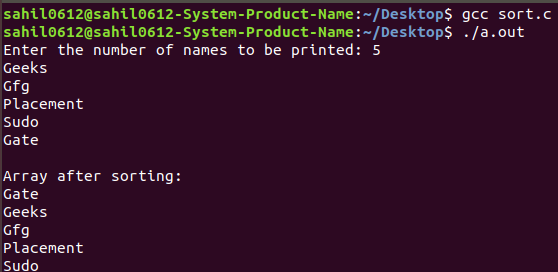# Sorting a dynamic 2-dimensional array of Strings

Prerequisite: How to dynamically allocate a 2D array in C?

Double pointer: A pointer pointing to another pointer is known as a Double pointer. To represent the double pointer ‘ ** ‘ is used. Double pointer is also called as pointer to pointer.

Example:

```Input: Geeks, Gfg,  Placement, Sudo, Gate
Output: Gate, Geeks, Gfg, Placement, Sudo
```

The idea is to dynamically allocate memory and values to the strings in a form of a 2-D array. Then apply bubble sort using strcmp and strcpy function.

Below is the required implementation:

 `// C program to sort an array of strings ` `#include ` `#include ` `#include ` ` `  `// Function to sort the values ` `void` `sort(``char``** names, ``int` `n) ` `{ ` `    ``int` `i, j; ` ` `  `    ``// Perform sort operation using bubble sort ` `    ``for` `(i = 0; i < n - 1; i++) ` `        ``for` `(j = 0; j < n - i - 1; j++) ` `            ``if` `(``strcmp``(names[j], names[j + 1]) > 0) { ` `                ``char``* temp; ` `                ``temp = (``char``*)``calloc``(30, ``sizeof``(``char``)); ` `                ``strcpy``(temp, names[j]); ` `                ``strcpy``(names[j], names[j + 1]); ` `                ``strcpy``(names[j + 1], temp); ` `            ``} ` `} ` ` `  `// Driver code ` `int` `main() ` `{ ` `    ``char``** names; ` `    ``int` `n, i; ` `    ``printf``(``"Enter the number of names to be printed: "``); ` `    ``scanf``(``"%d\n"``, &n); ` ` `  `    ``// allocating memory for 1st dimension ` `    ``names = (``char``**)``calloc``(n, ``sizeof``(``char``*)); ` ` `  `    ``for` `(i = 0; i < n; i++) ` `    ``// allocating memory for 2nd dimension ` `    ``{ ` `        ``names[i] = (``char``*)``calloc``(30, ``sizeof``(``char``)); ` `        ``scanf``(``"%s"``, names[i]); ` `    ``} ` `    ``sort(names, n); ` ` `  `    ``printf``(``"\nArray after sorting:\n"``); ` `    ``for` `(i = 0; i < n; i++) ` `        ``printf``(``"%s\n"``, names[i]); ` ` `  `    ``return` `0; ` `} `

Output:My Personal Notes arrow_drop_upCheck out this Author's contributed articles.

If you like GeeksforGeeks and would like to contribute, you can also write an article using contribute.geeksforgeeks.org or mail your article to contribute@geeksforgeeks.org. See your article appearing on the GeeksforGeeks main page and help other Geeks.

Please Improve this article if you find anything incorrect by clicking on the "Improve Article" button below.

Article Tags :
Practice Tags :

Be the First to upvote.

Please write to us at contribute@geeksforgeeks.org to report any issue with the above content.